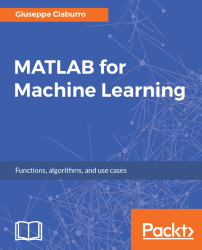•#### MATLAB for Machine Learning#### Overview of this book

MATLAB is the language of choice for many researchers and mathematics experts for machine learning. This book will help you build a foundation in machine learning using MATLAB for beginners. You’ll start by getting your system ready with t he MATLAB environment for machine learning and you’ll see how to easily interact with the Matlab workspace. We’ll then move on to data cleansing, mining and analyzing various data types in machine learning and you’ll see how to display data values on a plot. Next, you’ll get to know about the different types of regression techniques and how to apply them to your data using the MATLAB functions. You’ll understand the basic concepts of neural networks and perform data fitting, pattern recognition, and clustering analysis. Finally, you’ll explore feature selection and extraction techniques for dimensionality reduction for performance improvement. At the end of the book, you will learn to put it all together into real-world cases covering major machine learning algorithms and be comfortable in performing machine learning with MATLAB.
Title PageCreditsForewordwww.PacktPub.comCustomer FeedbackPrefaceFree Chapter
Getting Started with MATLAB Machine LearningImporting and Organizing Data in MATLABFrom Data to Knowledge DiscoveryFinding Relationships between Variables - Regression TechniquesPattern Recognition through Classification AlgorithmsIdentifying Groups of Data Using Clustering MethodsSimulation of Human Thinking - Artificial Neural NetworksImproving the Performance of the Machine Learning Model - Dimensionality ReductionMachine Learning in Practice## Chapter 7. Simulation of Human Thinking - Artificial Neural Networks

Artificial Neural Networks (ANN) are mathematical models for the simulation of typical human brain activities such as image perception, pattern recognition, language understanding, sense-motor coordination, and so on. These models are composed of a system of nodes, equivalent to the neurons of a human brain, which are interconnected by weighted links, equivalent to the synapses between neurons. The output of the network is modified iteratively from link weights to convergence. The original data is provided to the input layer and the result of the network is returned from the output level. The input nodes represent the independent or predictor variables that are used to predict the dependent variables, that is, the output neurons.

The MATLAB Neural Network Toolbox provides algorithms, pretrained models, and apps to create, train, visualize, and simulate artificial neural networks. We can perform classification, regression...